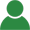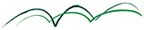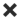ErrorHome Courses Course Calendar Teaching Team About Us Resources Contact
Course Calendar

# Volatility: Trading and Managing Risk

## Day One

### Black-Scholes Revisited

• A quick revision of Black-Scholes and Ito lemma
• Black-Scholes Greeks
• Black-Scholes implied volatility and implied risk neutral distributions
• Examples of derivatives sensitive to the whole volatility surface
• Motivation for alternatives to Black-Scholes and stochastic volatility

### Local Volatility

• Is Local Volatility a stochastic volatility model?
• Calculating a Local Volatility
• Implementing Local Volatility models
• Local Volatility as a conditional expectation of instantaneous volatility
• Weaknesses of Local Volatility models

Workshop: Calibrating local volatility and pricing a Barrier option

• Volatility Skew and Smile
• The Greeks
• Variance Swaps and Volatility Swaps

Workshop: Fitting a volatility surface and pricing a variance swap

## Day Two

### Heston and the Volatility Surface

• Looking at volatility dynamics in the real world
• The Heston equation
• The role of market price of volatility risk
• Volatility surface sensitivities to Heston parameters
• Linking Heston parameters to Black-Scholes implied volatilities
• Implication of the Heston volatility surface dynamics
• Simulating the Heston process

Workshop: Simulating the Heston dynamics and using it to price a Barrier option

### SABR and the Volatility Surface

• SABR: Stochastic Alpha, Beta and Rho
• SABR calibration
• SABR parameters and the volatility surface
• Sticky strike vs. sticky moneyness
• SABR in interest rate modelling and LMM-SABR

• Volatility indices – VIX and VSTOXX
• Volatility as an Asset Class – VXX and VXZ
• Incorporating Volatility into an Investment Portfolio
• Futures and Options on Volatility Indices
• The need for a stochastic volatility model
• Hedging Volatility Indices

Workshop: Finding a risk neutral distribution of volatility

## Day Three

### Market Models of Volatility

• Volatility surfaces revisited – extrapolation and interpolation
• Combining risk neutral distributions with a copula
• Using volatility smiles and copulae for pricing basket and spread options
• Market models of volatility options
• Arbitrage between volatility options and S&P options

Workshop: Relating VIX options and variance swaps

### Hedging Volatility Exposure

• Hedging volatility exposure of a book of exotic options
• Static vs. Dynamic Hedging
• Impact of Model choice
• Smile risk
• Understanding greeks
• Vega convexity

Workshop: Finding the best vega hedge